CBSE (Science) Class 11CBSE
Share

# HC Verma solutions for Class 11 Physics chapter 15 - Wave Motion and Waves on a String

#### HC Verma Concept of Physics Part-1 (2018-2019 Session) by H.C Verma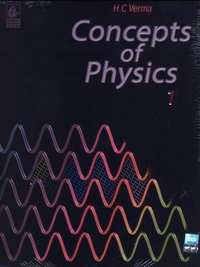## Chapter 15: Wave Motion and Waves on a String

#### HC Verma solutions for Class 11 Physics Chapter 15 Exercise Short Answers [Page 321]

Short Answers | Q 1 | Page 321

You are walking along a seashore and a mild wind is blowing. Is the motion of air a wave motion?

Short Answers | Q 2 | Page 321

The radio and TV programmes, telecast at the studio, reach our antenna by wave motion. Is it a mechanical wave or nonmechanical?

Short Answers | Q 3 | Page 321

A wave is represented by an equation $y = c_1 \sin \left( c_2 x + c_3 t \right)$ In which direction is the wave going? Assume that $c_1 , c_2$ $c_3$ are all positive.

Short Answers | Q 4 | Page 321

Show that the particle speed can never be equal to the wave speed in a sine wave if the amplitude is less than wavelength divided by 2π.

Short Answers | Q 5 | Page 321

Two wave pulses identical in shape but inverted with respect to each other are produced at the two ends of a stretched string. At an instant when the pulses reach the middle, the string becomes completely straight. What happens to the energy of the two pulses?

Short Answers | Q 6 | Page 321

Show that for a wave travelling on a string
$\frac{y_{max}}{\nu_{max}} = \frac{\nu_{max}}{\alpha_{max}},$

where the symbols have usual meanings. Can we use componendo and dividendo taught in algebra to write
$\frac{y_{max} + \nu_{max}}{\nu_{max} - \nu_{max}} = \frac{\nu_{max} + \alpha_{max}}{\nu_{max} - \alpha_{max}}?$

Short Answers | Q 7 | Page 321

What is the smallest positive phase constant which is equivalent to 7⋅5 π?

Short Answers | Q 8 | Page 321

A string clamped at both ends vibrates in its fundamental mode. Is there any position (except the ends) on the string which can be touched without disturbing the motion? What if the string vibrates in its first overtone?

#### HC Verma solutions for Class 11 Physics Chapter 15 Exercise MCQ [Pages 322 - 323]

MCQ | Q 1 | Page 322

A sine wave is travelling in a medium. The minimum distance between the two particles, always having same speed, is

• $\lambda/4$

• $\lambda/3$

• $\lambda/2$

• $\lambda$

MCQ | Q 2 | Page 322

A sine wave is travelling in a medium. A particular particle has zero displacement at a certain instant. The particle closest to it having zero displacement is at a distance

• $\lambda/4$

• $\lambda/3$

• $\lambda/2$

• $\lambda$

MCQ | Q 3 | Page 322

Which of the following equations represents a wave travelling along Y-axis?

• $x = A \sin \left( ky - \omega t \right)$

• $y = A \sin \left( kx - \omega t \right)$

• $y = A \sin ky \cos \omega t$

• $y = A \cos ky \sin \omega t$

MCQ | Q 4 | Page 322

The equation $y = A \sin^2 \left( kx - \omega t \right)$
represents a wave motion with

• amplitude A, frequency $\omega/2\pi$

• amplitude A/2, frequency $\omega/\pi$

• amplitude 2A, frequency $\omega/4\pi$

• does not represent a wave motion.

MCQ | Q 5 | Page 322

Which of the following is a mechanical wave?

•  X-rays

•  Light waves

• Sound waves.

MCQ | Q 6 | Page 322

A cork floating in a calm pond executes simple harmonic motion of frequency
$\nu$ when a wave generated by a boat passes by it. The frequency of the wave is

• $\nu$

• $\nu/2$

• $2\nu$

• $\sqrt{2}\nu$

MCQ | Q 7 | Page 322

Two strings A and B, made of same material, are stretched by same tension. The radius of string A is double of the radius of B. A transverse wave travels on A with speed $\nu_A$ and on B with speed $\nu_B$. The ratio $\nu_A / \nu_B$

• 1/2

• 2

•  1/4

• 4.

MCQ | Q 8 | Page 322

Both the strings, shown in figure, are made of same material and have same cross section. The pulleys are light. The wave speed of a transverse wave in the string AB is
$\nu_1$  and in CD it is $\nu_2$. Then $\nu_1 / \nu_2$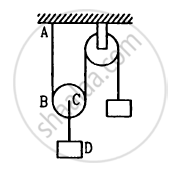• 1

• 2

• $\sqrt{2}$

• $1/\sqrt{2}$

MCQ | Q 9 | Page 322

Velocity of sound in air is 332 m s−1. Its velocity in vacuum will be

• > 332 m s1

•  = 332 m s−1

• < 332 m s−1

• meaningless.

MCQ | Q 10 | Page 322

A wave pulse, travelling on a two-piece string, gets partially reflected and partially transmitted at the junction. The reflected wave is inverted in shape as compared to the incident one. If the incident wave has wavelength λ and the transmitted wave λ'

• $\lambda' > \lambda$

• $\lambda' = \lambda$

• $\lambda' < \lambda$

• nothing can be said about the relation of $\lambda \text{ and } \lambda'$

MCQ | Q 11 | Page 322

Two waves represented by $y = a\sin\left( \omega t - kx \right)$ and $y = a\cos\left( \omega t - kx \right)$ $y = a\cos\left( \omega t - kx \right)$ are superposed. The resultant wave will have an amplitude

• a

• $\sqrt{2}a$

•  2a

• 0.

MCQ | Q 12 | Page 322

Two wires A and B, having identical geometrical construction, are stretched from their natural length by small but equal amount. The Young modules of the wires are YA and YB whereas the densities are $\rho_A \text{ and } \rho_B$. It is given that YA > YB and $\rho_A > \rho_B$. A transverse signal started at one end takes a time t1 to reach the other end for A and t2 for B.

•  t1 < t2

•  t1 = t2

• t1 > t2

• the information is insufficient to find the relation between t1 and t2.

MCQ | Q 13 | Page 322

Consider two waves passing through the same string. Principle of superposition for displacement says that the net displacement of a particle on the string is sum of the displacements produced by the two waves individually. Suppose we state similar principles for the net velocity of the particle and the net kinetic energy of the particle. Such a principle will be valid for

• both the velocity and the kinetic energy

• the velocity but not for the kinetic energy

• the kinetic energy but not for the velocity

•  neither the velocity nor the kinetic energy.

MCQ | Q 14 | Page 322

Two wave pulses travel in opposite directions on a string and approach each other. The shape of one pulse is inverted with respect to the other.

• The pulses will collide with each other and vanish after collision.

• The pulses will reflect from each other, i.e., the pulse going towards right will finally move towards left and vice versa.

• The pulses will pass through each other but their shapes will be modified.

• The pulses will pass through each other without any change in their shapes.

MCQ | Q 15 | Page 322

Two periodic waves of amplitudes A1 and A2 pass thorough a region. If A1 > A2, the difference in the maximum and minimum resultant amplitude possible is

• 2A1

• 2A2

•  A1 + A2

•  A1 − A2

MCQ | Q 16 | Page 322

Two waves of equal amplitude A, and equal frequency travel in the same direction in a medium. The amplitude of the resultant wave is

•  0

• A

•  2A

• between 0 and 2A.

MCQ | Q 17 | Page 322

Two sine waves travel in the same direction in a medium. The amplitude of each wave is A and the phase difference between the two waves is 120°. The resultant amplitude will be

•  A

•  2A

• 4A

• $\sqrt{2}A$

MCQ | Q 18 | Page 322

The fundamental frequency of a string is proportional to

• inverse of its length

• the diameter

•  the tension

• the density.

MCQ | Q 19 | Page 323

A tuning fork of frequency 480 Hz is used to vibrate a sonometer wire having natural frequency 240 Hz. The wire will vibrate with a frequency of

•  240 Hz

• 480 Hz

•  720 Hz

• will not vibrate.

MCQ | Q 20 | Page 323

A tuning fork of frequency 480 Hz is used to vibrate a sonometer wire having natural frequency 410 Hz. The wire will vibrate with a frequency

• 410 Hz

• 480 Hz

• 820 Hz

• 960 Hz.

MCQ | Q 21 | Page 323

A sonometer wire of length l vibrates in fundamental mode when excited by a tuning fork of frequency 416. Hz. If the length is doubled keeping other things same, the string will

•  vibrate with a frequency of 416 Hz

•  vibrate with a frequency of 208 Hz

•  vibrate with a frequency of 832 Hz

• stop vibrating.

MCQ | Q 22 | Page 323

A sonometer wire supports a 4 kg load and vibrates in fundamental mode with a tuning fork of frequency 416. Hz. The length of the wire between the bridges is now doubled. In order to maintain fundamental mode, the load should be changed to

• 1 kg

• 2 kg

• 8 kg

• 16 kg.

#### HC Verma solutions for Class 11 Physics Chapter 15 Exercise MCQ [Page 323]

MCQ | Q 1 | Page 323

A mechanical wave propagates in a medium along the X-axis. The particles of the medium
(a) must move on the X-axis
(b) must move on the Y-axis
(c) may move on the X-axis
(d) may move on the Y-axis.

MCQ | Q 2 | Page 323

A transverse wave travels along the Z-axis. The particles of the medium must move

•  along the Z-axis

• along the X-axis

• along the Y-axis

• in the X-Y plane.

MCQ | Q 3 | Page 323

Longitudinal waves cannot

• have a unique wavelength

• transmit energy

• have a unique wave velocity

• be polarized.

MCQ | Q 4 | Page 323

A wave going in a solid
(a) must be longitudinal
(b) may be longitudinal
(c) must be transverse
(d) may be transverse.

MCQ | Q 5 | Page 323

A wave moving in a gas

• must be longitudinal

• may be longitudinal

• must transverse

• may be transverse.

MCQ | Q 6 | Page 323

Two particles A and B have a phase difference of π when a sine wave passes through the region.
(a) A oscillates at half the frequency of B.
(b) A and B move in opposite directions.
(c) A and B must be separated by half of the wavelength.
(d) The displacements at A and B have equal magnitudes.

MCQ | Q 7 | Page 323

A wave is represented by the equation
$y = \left( 0 \text{ cdot 001 mm }\right) \sin\left[ \left( 50 s^{- 1} \right)t + \left( 2 \cdot 0 m^{- 1} \right)x \right]$
(a) The wave velocity = 100 m s−1.
(b) The wavelength = 2⋅0 m.
(c) The frequency = 25/π Hz.
(d) The amplitude = 0⋅001 mm.

MCQ | Q 8 | Page 323

A standing wave is produced on a string clamped at one end and free at the other. The length of the string

• must be an integral multiple of $\lambda/4$

• must be an integral multiple of $\lambda/2$

• must be an integral multiple of $\lambda/2$

• must be an integral multiple of $\lambda$

MCQ | Q 9 | Page 323

Mark out the correct options.

• The energy of any small part of a string remains constant in a travelling wave.

• The energy of any small part of a string remains constant in a standing wave.

•  The energies of all the small parts of equal length are equal in a travelling wave.

• The energies of all the small parts of equal length are equal in a standing wave.

MCQ | Q 10 | Page 323

In a stationary wave,
(a) all the particles of the medium vibrate in phase
(b) all the antinodes vibrates in phase
(c) the alternate antinodes vibrate in phase
(d) all the particles between consecutive nodes vibrate in phase.

#### HC Verma solutions for Class 11 Physics Chapter 15 [Pages 323 - 327]

Q 1 | Page 323

A wave pulse passing on a string with a speed of 40 cm s−1 in the negative x-direction has its maximum at x = 0 at t = 0. Where will this maximum be located at t = 5 s?

Q 2 | Page 323

The equation of a wave travelling on a string stretched along the X-axis is given by
$y = A e {}^- \left( \frac{x}{a} + \frac{t}{T} \right)^2 .$
(a) Write the dimensions of A, a and T. (b) Find the wave speed. (c) In which direction is the wave travelling? (d) Where is the maximum of the pulse located at t = T? At t = 2 T?

Q 3 | Page 323

following Figure shows a wave pulse at t = 0. The pulse moves to the right with a speed of 10 cm s−1. Sketch the shape of the string at t = 1 s, 2 s and 3 s.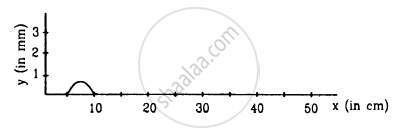Q 4 | Page 324

A pulse travelling on a string is represented by the function $y = \frac{a^2}{\left( x - \nu t \right)^2 + a^2},$ where a = 5 mm and ν = 20 cm-1. Sketch the shape of the string at t = 0, 1 s and 2 s. Take x = 0 in the middle of the string.

Q 5 | Page 324

The displacement of the particle at x = 0 of a stretched string carrying a wave in the positive x-direction is given f(t) = A sin (t/T). The wave speed is  v. Write the wave equation.

Q 6 | Page 324

A wave pulse is travelling on a string with a speed $\nu$ towards the positive X-axis. The shape of the string at t = 0 is given by g(x) = Asin(x/a), where A and a are constants. (a) What are the dimensions of A and a ? (b) Write the equation of the wave for a general time t, if the wave speed is $\nu$.

Q 7 | Page 324

A wave propagates on a string in the positive x-direction at a velocity $\nu$ $t = t_0$ is given by $g\left( x, t_0 \right) = A \sin \left( x/a \right)$. Write the wave equation for a general time t.

Q 8 | Page 324

The equation of a wave travelling on a string is $y = \left( 0 \cdot 10 \text{ mm } \right) \sin\left[ \left( 31 \cdot 4 m^{- 1} \right)x + \left( 314 s^{- 1} \right)t \right]$
(a) In which direction does the wave travel? (b) Find the wave speed, the wavelength and the frequency of the wave. (c) What is the maximum displacement and the maximum speed of a portion of the string?

Q 9 | Page 324

A wave travels along the positive x-direction with a speed of 20 m s−1. The amplitude of the wave is 0⋅20 cm and the wavelength 2⋅0 cm. (a) Write the suitable wave equation which describes this wave. (b) What is the displacement and velocity of the particle at x= 2⋅0 cm at time = 0 according to the wave equation written? Can you get different values of this quantity if the wave equation is written in a different fashion?

Q 10 | Page 324

A wave is described by the equation $y = \left( 1 \cdot 0 mm \right) \sin \pi\left( \frac{x}{2 \cdot 0 cm} - \frac{t}{0 \cdot 01 s} \right) .$
(a) Find the time period and the wavelength? (b) Write the equation for the velocity of the particles. Find the speed of the particle at x = 1⋅0 cm at time t = 0⋅01 s. (c) What are the speeds of the particles at x = 3⋅0 cm, 5⋅0 cm and 7⋅0 cm at t = 0⋅01 s?
(d) What are the speeds of the particles at x = 1⋅0 cm at t = 0⋅011, 0⋅012, and 0⋅013 s?

Q 11 | Page 324

A particle on a stretched string supporting a travelling wave, takes 5⋅0 ms to move from its mean position to the extreme position. The distance between two consecutive particles, which are at their mean positions, is 2⋅0 cm. Find the frequency, the wavelength and the wave speed.

Q 12 | Page 324

Figure shows a plot of the transverse displacements of the particles of a string at t = 0 through which a travelling wave is passing in the positive x-direction. The wave speed is 20 cm s−1. Find (a) the amplitude, (b) the wavelength, (c) the wave number and (d) the frequency of the wave.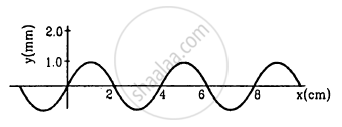Q 13 | Page 324

A wave travelling on a string at a speed of 10 m s−1 causes each particle of the string to oscillate with a time period of 20 ms. (a) What is the wavelength of the wave? (b) If the displacement of a particle of 1⋅5 mm at a certain instant, what will be the displacement of a particle 10 cm away from it at the same instant?

Q 14 | Page 324

A steel wire of length 64 cm weighs 5 g. If it is stretched by a force of 8 N, what would be the speed of a transverse wave passing on it?

Q 15 | Page 324

A string of length 20 cm and linear mass density 0⋅40 g cm−1 is fixed at both ends and is kept under a tension of 16 N. A wave pulse is produced at t = 0 near an ends as shown in the figure, which travels towards the other end. (a) When will the string have the shape shown in the figure again? (b) Sketch the shape of the string at a time half of that found in part (a).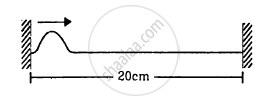Q 16 | Page 324

A string of linear mass density 0⋅5 g cm−1 and a total length 30 cm is tied to a fixed wall at one end and to a frictionless ring at the other end (See figure). The ring can move on a vertical rod. A wave pulse is produced on the string which moves towards the ring at a speed of 20 cm s−1. The pulse is symmetric about its maximum which is located at a distance of 20 cm from the end joined to the ring. (a) Assuming that the wave is reflected from the ends without loss of energy, find the time taken by the string to region its shape. (b) The shape of the string changes periodically with time. Find this time period. (c) What is the tension in the string?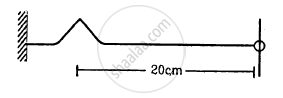Q 17 | Page 324

Two wires of different densities but same area of cross section are soldered together at one end and are stretched to a tension T. The velocity of a transverse wave in the first wire is double of that in the second wire. Find the ratio of the density of the first wire to that of the second wire.

Q 18 | Page 324

A transverse wave described by $y = \left( 0 \cdot 02 m \right) \sin \left( 1 \cdot 0 m^{- 1} \right) x + \left( 30 s^{- 1} \right)t$ propagates on a stretched string having a linear mass density of $1 \cdot 2 \times {10}^{- 4} kg m^{- 1}$ the tension in the string.

Q 19 | Page 325

A travelling wave is produced on a long horizontal string by vibrating an end up and down sinusoidally. The amplitude of vibration is 1⋅0 and the displacement becomes zero 200 times per second. The linear mass density of the string is 0⋅10 kg m−1 and it is kept under a tension of 90 N. (a) Find the speed and the wavelength of the wave. (b) Assume that the wave moves in the positive x-direction and at t = 0, the end x = 0 is at its positive extreme position. Write the wave equation. (c) Find the velocity and acceleration of the particle at x = 50 cm at time t = 10 ms.

Q 20 | Page 324

A string of length 40 cm and weighing 10 g is attached to a spring at one end and to a fixed wall at the other end. The spring has a spring constant of 160 N m−1 and is stretched by 1⋅0 cm. If a wave pulse is produced on the string near the wall, how much time will it take to reach the spring?

Q 21 | Page 325

Two blocks each having a mass of 3⋅2 kg are connected by a wire CD and the system is suspended from the ceiling by another wire AB (See following figure). The linear mass density of the wire AB is 10 g m−1 and that of CD is 8 g m−1. Find the speed of a transverse wave pulse produced in AB and CD.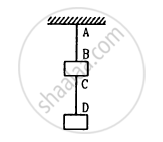Q 22 | Page 325

In the arrangement shown in figure  , the string has a mass of 4⋅5 g. How much time will it take for a transverse disturbance produced at the floor to reach the pulley? Take g = 10 m s−2.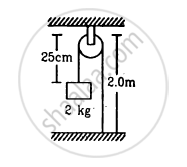Q 23 | Page 325

A 4⋅0 kg block is suspended from the ceiling of an elevator through a string having a linear mass density of $19 \cdot 2 \times {10}^{- 3} kg m^{- 1}$  . Find the speed (with respect to the string) with which a wave pulse can proceed on the string if the elevator accelerates up at the rate of 2⋅0 m s−2. Take g = 10 m s−2.

Q 24 | Page 325

A heavy ball is suspended from the ceiling of a motor car through a light string. A transverse pulse travels at a speed of 60 cm s −1 on the string when the car is at rest and 62 cm s−1 when the car accelerates on a horizontal road. Find the acceleration of the car. Take g = 10 m s−2

Q 25 | Page 325

A circular loop of string rotates about its axis on a frictionless horizontal place at a uniform rate so that the tangential speed of any particle of the string is ν.  If a small transverse disturbance is produced at a point of the loop, with what speed (relative to the string) will this disturbance travel on the string?

Q 26 | Page 325

A heavy but uniform rope of length L is suspended from a ceiling. (a) Write the velocity of a transverse wave travelling on the string as a function of the distance from the lower end. (b) If the rope is given a sudden sideways jerk at the bottom, how long will it take for the pulse to reach the ceiling? (c) A particle is dropped from the ceiling at the instant the bottom end is given the jerk. Where will the particle meet the pulse?

Q 27 | Page 325

Two long strings A and B, each having linear mass density
$1 \cdot 2 \times {10}^{- 2} kg m^{- 1}$ , are stretched by different tensions 4⋅8 N and 7⋅5 N respectively and are kept parallel to each other with their left ends at x = 0. Wave pulses are produced on the strings at the left ends at t = 0 on string A and at t = 20 ms on string B. When and where will the pulse on B overtake that on A?

Q 28 | Page 325

A transverse wave of amplitude 0⋅50 mm and frequency 100 Hz is produced on a wire stretched to a tension of 100 N. If the wave speed is 100 m s−1, what average power is the source transmitting to the wire?

Q 29 | Page 325

A 200 Hz wave with amplitude 1 mm travels on a long string of linear mass density 6 g m−1 kept under a tension of 60 N. (a) Find the average power transmitted across a given point on the string. (b) Find the total energy associated with the wave in a 2⋅0 m long portion of the string.

Q 30 | Page 325

A tuning fork of frequency 440 Hz is attached to a long string of linear mass density 0⋅01 kg m−1 kept under a tension of 49 N. The fork produces transverse waves of amplitude 0⋅50 mm on the string. (a) Find the wave speed and the wavelength of the waves. (b) Find the maximum speed and acceleration of a particle of the string. (c) At what average rate is the tuning fork transmitting energy to the string?

Q 31 | Page 325

Two waves, travelling in the same direction through the same region, have equal frequencies, wavelengths and amplitudes. If the amplitude of each wave is 4 mm and the phase difference between the waves is 90°, what is the resultant amplitude?

Q 32 | Page 325

Following figure shows two wave pulses at t = 0 travelling on a string in opposite directions with the same wave speed 50 cm s−1. Sketch the shape of the string at t = 4 ms, 6 ms, 8 ms, and 12 ms.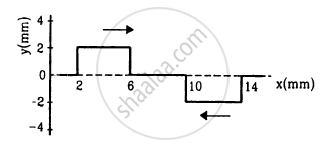Q 33 | Page 326

Two waves, each having a frequency of 100 Hz and a wavelength of 2⋅0 cm, are travelling in the same direction on a string. What is the phase difference between the waves (a) if the second wave was produced 0⋅015 s later than the first one at the same place, (b) if the two waves were produced at the same instant but first one was produced a distance 4⋅0 cm behind the second one? (c) If each of the waves has an amplitude of 2⋅0 mm, what would be the amplitudes of the resultant waves in part (a) and (b) ?

Q 34 | Page 326

If the speed of a transverse wave on a stretched string of length 1 m is 60 m−1, what is the fundamental frequency of vibration?

Q 35 | Page 326

A wire of length 2⋅00 m is stretched to a tension of 160 N. If the fundamental frequency of vibration is 100 Hz, find its linear mass density.

Q 36 | Page 326

A steel wire of mass 4⋅0 g and length 80 cm is fixed at the two ends. The tension in the wire is 50 N. Find the frequency and wavelength of the fourth harmonic of the fundamental.

Q 37 | Page 326

A piano wire weighing 6⋅00 g and having a length of 90⋅0 cm emits a fundamental frequency corresponding to the "Middle C" $\left( \nu = 261 \cdot 63 Hz \right)$. Find the tension in the wire.

Q 38 | Page 326

A sonometer wire having a length of 1⋅50 m between the bridges vibrates in its second harmonic in resonance with a tuning fork of frequency 256 Hz. What is the speed of the transverse wave on the wire?

Q 39 | Page 326

The length of the wire shown in figure between the pulley is 1⋅5 m and its mass is 12⋅0 g. Find the frequency of vibration with which the wire vibrates in two loops leaving the middle point of the wire between the pulleys at rest.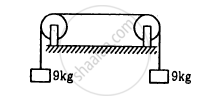Q 40 | Page 326

A one-metre long stretched string having a mass of 40 g is attached to a tuning fork. The fork vibrates at 128 Hz in a direction perpendicular to the string. What should be the tension in the string if it is to vibrate in four loops?

Q 41 | Page 326

A wire, fixed at both ends is seen to vibrate at a resonant frequency of 240 Hz and also at 320 Hz. (a) What could be the maximum value of the fundamental frequency? (b) If transverse waves can travel on this string at a speed of 40 m s−1, what is its length?

Q 42 | Page 326

A string, fixed at both ends, vibrates in a resonant mode with a separation of 2⋅0 cm between the consecutive nodes. For the next higher resonant frequency, this separation is reduced to 1⋅6 cm. Find the length of the string.

Q 43 | Page 326

A 660 Hz tuning fork sets up vibration in a string clamped at both ends. The wave speed for a transverse wave on this string is 220 m s−1 and the string vibrates in three loops. (a) Find the length of the string. (b) If the maximum amplitude of a particle is 0⋅5 cm, write a suitable equation describing the motion.

Q 44 | Page 326

A particular guitar wire is 30⋅0 cm long and vibrates at a frequency of 196 Hz when no finger is placed on it. The next higher notes on the scale are 220 Hz, 247 Hz, 262 Hz and 294 Hz. How far from the end of the string must the finger be placed to play these notes?

Q 45 | Page 326

A steel wire fixed at both ends has a fundamental frequency of 200 Hz. A person can hear sound of maximum frequency 14 kHz. What is the highest harmonic that can be played on this string which is audible to the person?

Q 46 | Page 326

Three resonant frequencies of a string are 90, 150 and 210 Hz. (a) Find the highest possible fundamental frequency of vibration of this string. (b) Which harmonics of the fundamental are the given frequencies? (c) Which overtones are these frequencies? (d) If the length of the string is 80 cm, what would be the speed of a transverse wave on this string?

Q 47 | Page 326

Two wires are kept tight between the same pair of supports. The tensions in the wires are in the ratio 2 : 1 the radii are in the ratio 3 : 1 and the densities are in the ratio 1 : 2. Find the ratio of their fundamental frequencies.

Q 48 | Page 326

A uniform horizontal rod of length 40 cm and mass 1⋅2 kg is supported by two identical wires as shown in figure. Where should a mass of 4⋅8 kg be placed on the rod so that the same tuning fork may excite the wire on left into its fundamental vibrations and that on right into its first overtone? Take g = 10 m s−2.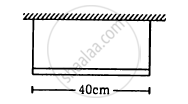Q 49 | Page 326

Figure shows an aluminium wire of length 60 cm joined to a steel wire of length 80 cm and stretched between two fixed supports. The tension produced is 40 N. The cross-sectional area of the steel wire is 1⋅0 mm2 and that of the aluminium wire is 3⋅0 mm2. What could be the minimum frequency of a tuning fork which can produce standing waves in the system with the joint as a node? The density of aluminium is 2⋅6 g cm−3 and that of steel is 7⋅8 g cm−3.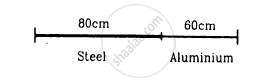Q 50 | Page 326

A string of length L fixed at both ends vibrates in its fundamental mode at a frequency ν and a maximum amplitude A. (a) Find the wavelength and the wave number k. (b). Take the origin at one end of the string and the X-axis along the string. Take the Y-axis along the direction of the displacement. Take t = 0 at the instant when the middle point of the string passes through its mean position and is going towards the positive y-direction. Write the equation describing the standing wave.

Q 51 | Page 327

A 2 m long string fixed at both ends is set into vibrations in its first overtone. The wave speed on the string is 200 m s−1 and the amplitude is 0⋅5 cm. (a) Find the wavelength and the frequency. (b) Write the equation giving the displacement of different points as a function of time. Choose the X-axis along the string with the origin at one end and  t = 0 at the instant when the point x = 50 cm has reached its maximum displacement.

Q 52 | Page 327

The equation for the vibration of a string, fixed at both ends vibrating in its third harmonic, is given by
$y = \left( 0 \cdot 4 cm \right) \sin\left[ \left( 0 \cdot 314 {cm}^{- 1} \right) x \right] \cos \left[ \left( 600\pi s^{- 1} \right) t \right]$
(a) What is the frequency of vibration? (b) What are the positions of the nodes? (c) What is the length of the string? (d) What is the wavelength and the speed of two travelling waves that can interfere to give this vibration?

Q 53 | Page 327

The equation of a standing wave, produced on a string fixed at both ends, is
$y = \left( 0 \cdot 4 cm \right) \sin \left[ \left( 0 \cdot 314 {cm}^{- 1} \right) x \right] \cos \left[ \left( 600\pi s^{- 1} \right) t \right]$
What could be the smallest length of the string?

Q 54 | Page 327

A 40 cm wire having a mass of 3⋅2 g is stretched between two fixed supports 40⋅05 cm apart. In its fundamental mode, the wire vibrates at 220 Hz. If the area of cross section of the wire is 1⋅0 mm2, find its Young modulus.

Q 55 | Page 327

Following figure shows a string stretched by a block going over a pulley. The string vibrates in its tenth harmonic in unison with a particular tuning for. When a beaker containing water is brought under the block so that the block is completely dipped into the beaker, the string vibrates in its eleventh harmonic. Find the density of the material of the block.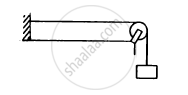Q 56 | Page 327

A 2⋅00 m-long rope, having a mass of 80 g, is fixed at one end and is tied to a light string at the other end. The tension in the string is 256 N. (a) Find the frequencies of the fundamental and the first two overtones. (b) Find the wavelength in the fundamental and the first two overtones.

Q 57 | Page 327

A heavy string is tied at one end to a movable support and to a light thread at the other end as shown in following figure. The thread goes over a fixed pulley and supports a weight to produce a tension. The lowest frequency with which the heavy string resonates is 120 Hz. If the movable support is pushed to the right by 10 cm so that the joint is placed on the pulley, what will be the minimum frequency at which the heavy string can resonate?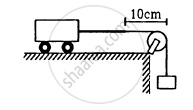## Chapter 15: Wave Motion and Waves on a String

#### HC Verma Concept of Physics Part-1 (2018-2019 Session) by H.C Verma## HC Verma solutions for Class 11 Physics chapter 15 - Wave Motion and Waves on a String

HC Verma solutions for Class 11 Physics chapter 15 (Wave Motion and Waves on a String) include all questions with solution and detail explanation. This will clear students doubts about any question and improve application skills while preparing for board exams. The detailed, step-by-step solutions will help you understand the concepts better and clear your confusions, if any. Shaalaa.com has the CBSE Concept of Physics Part-1 (2018-2019 Session) by H.C Verma solutions in a manner that help students grasp basic concepts better and faster.

Further, we at Shaalaa.com provide such solutions so that students can prepare for written exams. HC Verma textbook solutions can be a core help for self-study and acts as a perfect self-help guidance for students.

Concepts covered in Class 11 Physics chapter 15 Wave Motion and Waves on a String are Speed of Wave Motion, Reflection of Transverse and Longitudinal Waves, Displacement Relation for a Progressive Wave, The Speed of a Travelling Wave, Principle of Superposition of Waves,, Introduction of Reflection of Waves, Standing Waves and Normal Modes, Beats, Doppler Effect, Wave Motion.

Using HC Verma Class 11 solutions Wave Motion and Waves on a String exercise by students are an easy way to prepare for the exams, as they involve solutions arranged chapter-wise also page wise. The questions involved in HC Verma Solutions are important questions that can be asked in the final exam. Maximum students of CBSE Class 11 prefer HC Verma Textbook Solutions to score more in exam.

Get the free view of chapter 15 Wave Motion and Waves on a String Class 11 extra questions for Physics and can use Shaalaa.com to keep it handy for your exam preparation

S